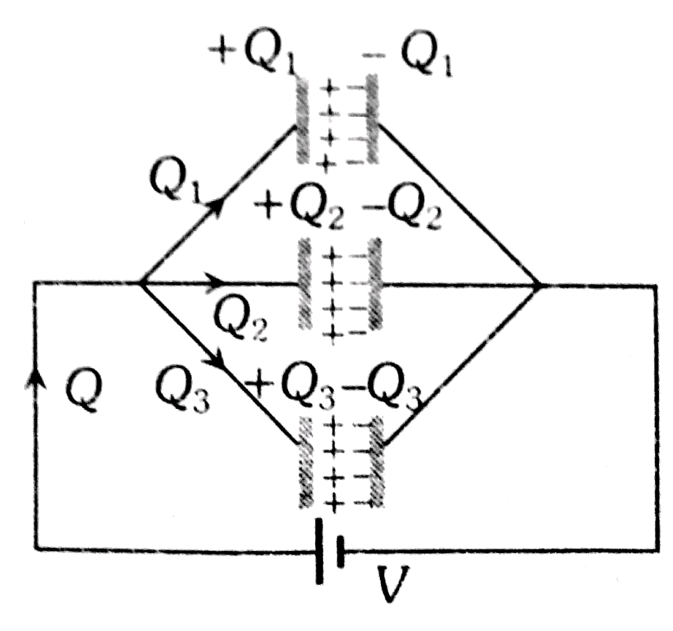# Two condensers of capacity C and 2C are connected in parallel and these are charged upto V volt. If the battery is removed and dielectric medium of constant K is put between the plates of first condenser, then the potential at each condenser is  Option 1) Option 2) Option 3) Option 4)

H Himanshu

As we have learned

Parallel Grouping -

- whereinInitially                 Equivalent capacitance of the system

Total charge  Q= (3C)V

Finally                 Equivalent capacitance of the system

Hence common potentialOption 1)

Option 2)

Option 3)

Option 4)

Exams
Articles
Questions